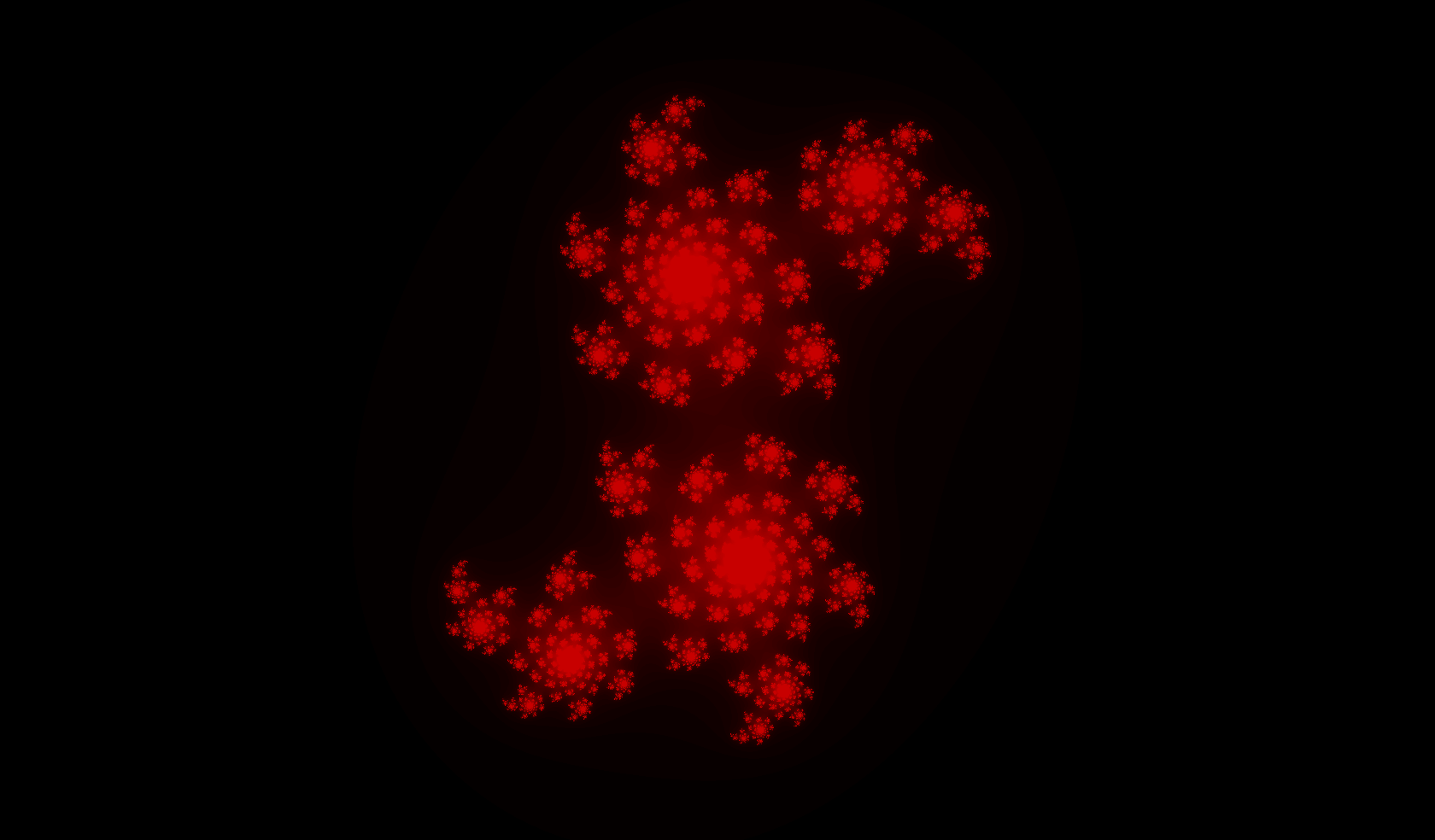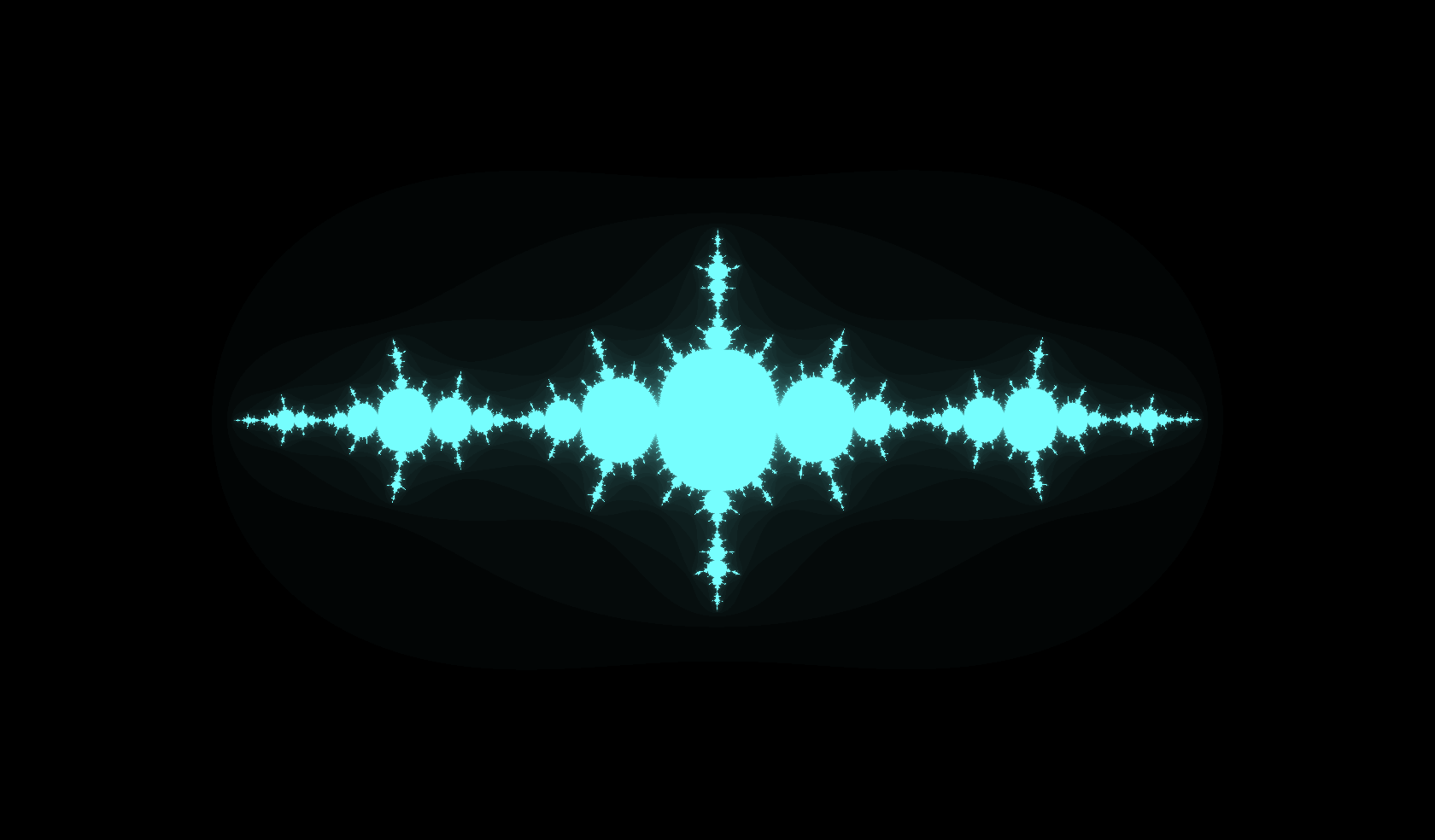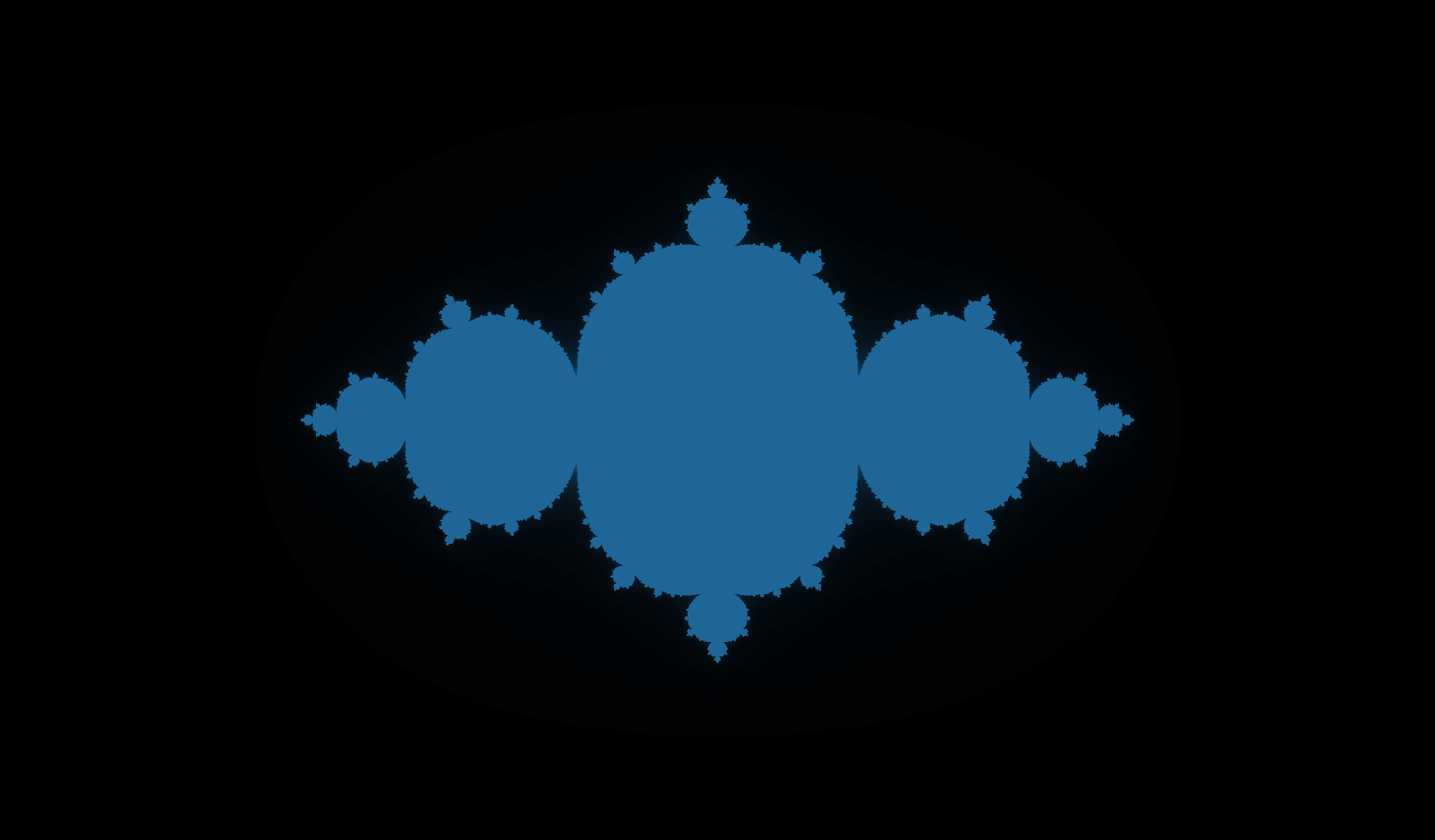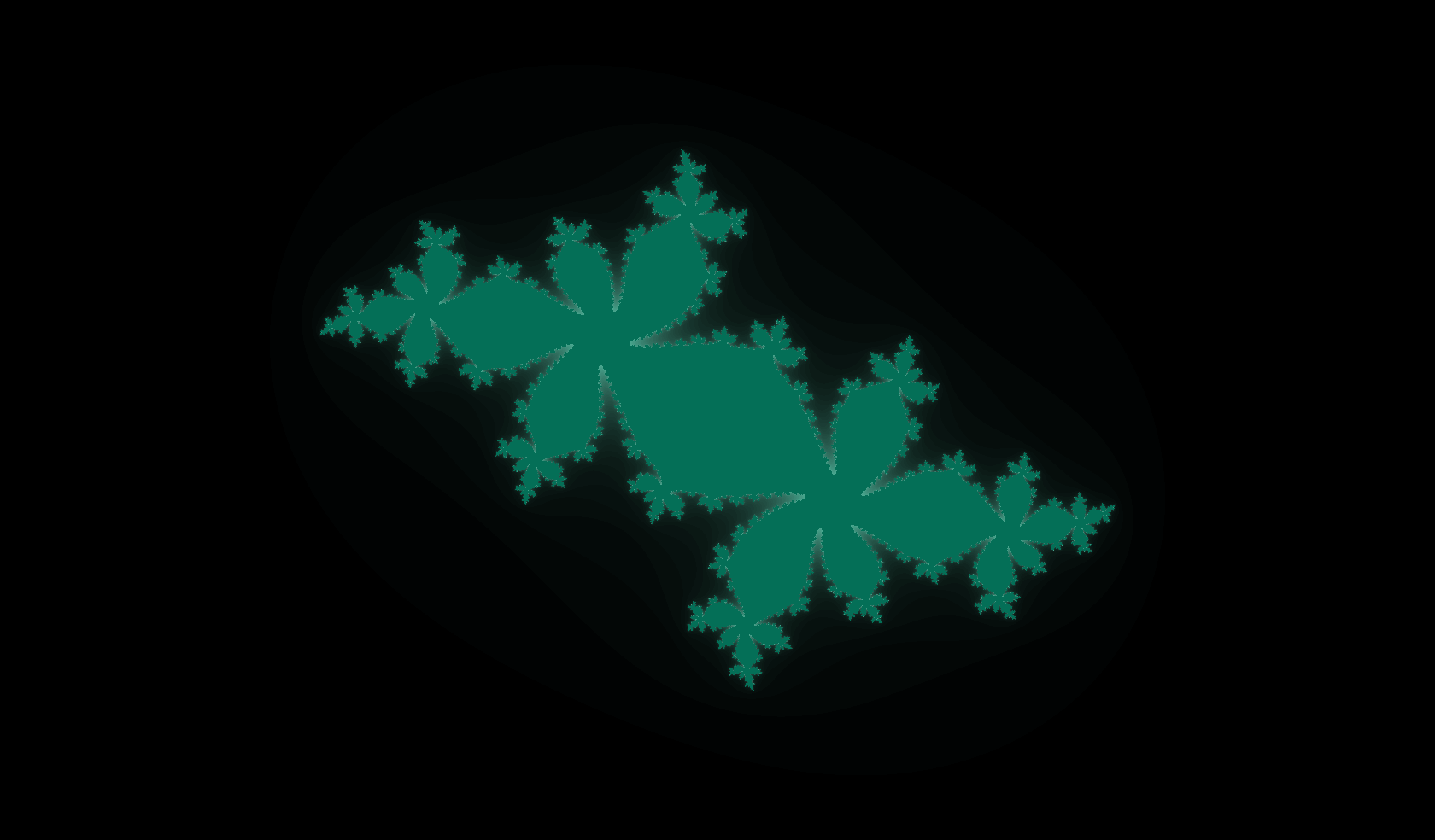# Mr. Julia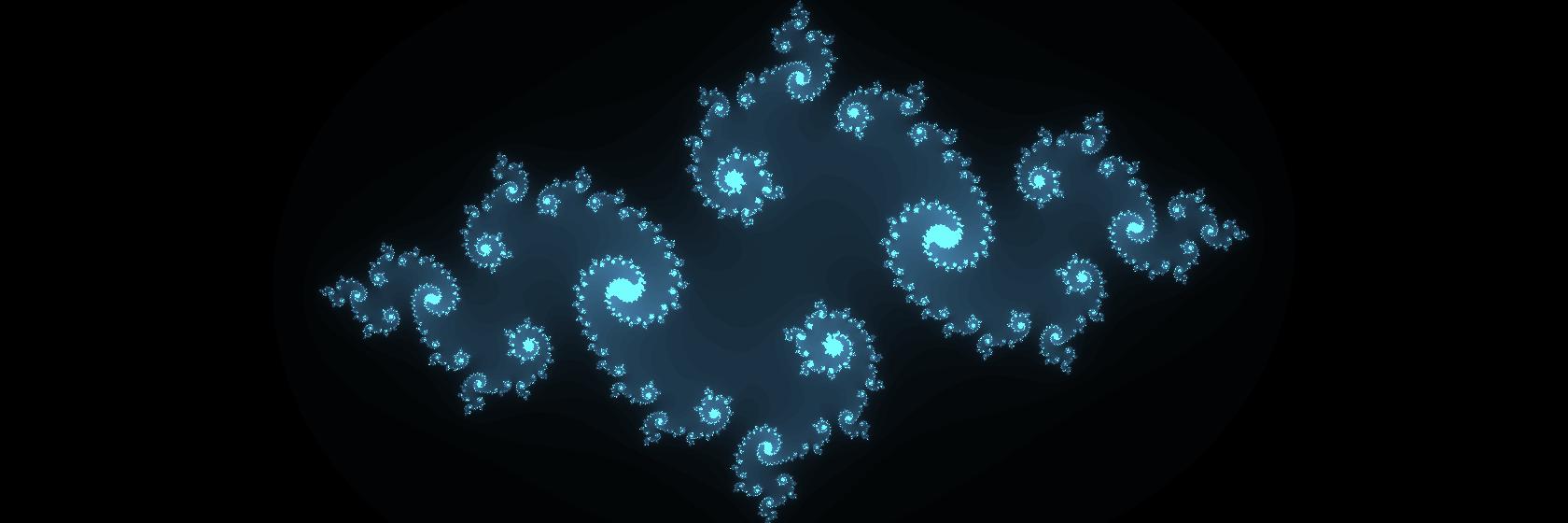Going on my theme of wonderfully fractal images, I wrote a little simulation to introduce myself to webGL. Go have a wee play about with it here.

### The Maths

You can find lots of information about Julia fractals all around the web so I won’t go into much detail at all here. All I’ll say is that the fractals, named for Gaston Julia, come about by iterating a complex number through the formula $$z_{n+1} = z_n^2 + c$$, where $$c$$ is some complex number. There’s no need to take the square but it produces some very nice images without going into the complexity of trying to take powers of complex numbers.

The pictures are produced by taking each pixel, turning its location ($$x, y$$) into a location in the complex plane $$z = x+ iy$$ and using that as the starting point for the iteration. The location of the mouse on the screen gives the value of $$c$$ and changes the entire nature of the fractal. Take a look at some of the fascinating patterns you can get below.

### Examples